# The scalar replacement theorem.

Ok ok I was a bit lazy but it is finished now so let’s finally post this scalar replacement theorem. Never in this post I formulate or proof this scalar replacement theorem, but basically this theorem says that if you replace the real numbers (scalars) in the way you describe say 2D split complex numbers by numbers from the complex plane, the result is a space who’s numbers also commute and it even has viable Cauchy Riemann equations. In this post I will write z = x + yj for the 2D circular numbers (also known as the split complex numbers) and write z = x + yi for numbers from the complex plane. If you combine such spaces it must have imaginary units that are different in notation, so j is the imaginary unit that does j^2 = 1 while the good ol i from the complex plane is known for it’s important property that i^2 = -1.

If we replace the x and y in z = x + yj by complex numbers we get a new 4D space where both j and i place there role. All in all those 4D numbers will be written as Z = a + bi + cj + dij. Of course the a, b, c and d are real numbers and as such this new space is 4D.

A long time ago I once used this to calculate the logatithm of j, it worked perfectly and that is why I more or less gave idea’s like that the name of ‘scalar replacement’. Later I found that way of using diagonalization of the matrix representations in order to calculate the logarithm, that is a far more general useable way of calculating logarithms but anyway the original calculation for log j was so cute, I could not abondon it and say to that calculation: From now on you are a poor orphan and no one will help you survive from day to day… How could I abandon such a calculation, better loose the UK a 100 times on a row than abandoning such nice calculations… 😉

But let’s go back to being a serious and responsible adult; the post is relatively long with 10 pictures. As usual I had to leave a lot out and I hope it is more or less easily readable. After all a lot of math out there looks like it is written by people who eat a plate of coal for breakfast. And if you eat coal for breakfast, likely this has an influence on the math you will produce on a particular day… Ok, here we go:

Ok, the goal of this post is of course to make you think a little bit about this 4D space and compare it to the quaternions and stuff. But last year on 2 March I posted the diagonalization method for finding the logarithm of an arbitrary split complex number. Below is a link.

Let me end this post with a funny mathematical joke about how to NOT WRITE MATH. Using a fucking lot of indices is not a way to make your work readable, here is a picture of what I view as some kind of mathematical joke.

https://arxiv.org/pdf/1906.09014.pdf

Ok, end of this post.

# Using the Cayley-Hamilton theorem to find ‘all’ multiplications in 3D space.

It is a bit vague what exactly a multiplication is, but I always use things that ‘rotate over the dimensions’. For example on the 3D complex space the imiginary unit is written as j and the powers of j simply rotate over the dimensions because:

j = (0, 1, 0)
j^2 = (0, 0, 1) and
j^3 = (-1, 0, 0). Etc, the period becomes 6 in this way because after the sixth power everything repeats.

In this post we will look at a more general formulation of what the third power of j is. The Cayley-Hamilton theorem says that you can write the third power of 3 by 3 matrices always as some linear combination of the lower powers.

That is what we do in this post; we take a look at j^3 = a + bj + cj^2. Here the a, b and c are real numbers. The allowed values that j^3 can take is what I call the ‘parameter space’. This parameter space is rather big, it is almost 3D real space but if you want the 3D Cauchy-Riemann equations to fly it has to be that a is always non zero. There is nothing mysterious about that demand of being non zero: if the constant a = 0, the imaginary unit is no longer invertible and that is the root cause of a whole lot of trouble and we want to avoid that.

It is well known that sir Hamilton tried to find the 3D complex numbers for about a full decade. Because he wanted this 3D complex number space as some extension of the complex plane, he failed in this detail and instead found the quaternions… But if the 3D numbers were some extension of the 2D complex plane, there should be at least one number X in 3D such that it squares to minus one. At the end I give a simple proof why the equation X^2 = -1 cannot be solved in 3D space for all allowed parameters. So although we have a 3D ocean of parameters and as such an infinite amount of different multiplications, none of them contains a number that squares to minus one…

I gave a small theorem covering the impossibility of solving X^2 = -1 a relative harsh name: Trashing the Hamilton approach for 3D complex numbers. This should not be viewed as some emotional statement about the Hamilton guy. It is just what it says: trashing that kind of approach…

This post is 7 pictures long, each of the usual size of 550×775 pixels.

Sorry for the test picture, but the seven jpg pictures refused to upload. And that is strange because they are just seven clean jpg’s. Now it is repaired although I do not understand this strange error.

Anyway have a cool summer. Till updates.

# Part 8 of the 4D basics: Wirtinger derivatives.

This is the 91-th post on this website so surely but slowly this website is growing on. This post was more or less written just for myself; I don’t know if the concept of Wirtinger derivates is used a lot in standard complex analysis but I sure like it so that’s why we take a look at it.

The idea of a Wirtinger derivative is very simple to understand: You have some function f(Z) and by differentiating it in the direction of all four basis vectors you craft the derivative f'(Z) from that.

At the basis for all the calculations we do in this post are the Cauchy-Riemann equations that allow you to rewrite the partial derivatives we put into the Wirtinger derivative.

The main result in this post is as follows:
We take our Wirtinger operator W and we multiply it with the 4D complex conjugate of W and we show that this is a real multiple of the Laplacian.

The 4D case is more or less the same as on the complex plane, that is not a miracle because in previous 4D basics we already observed two planes inside the 4D complex numbers that are isomorphic to the complex plane. So it is not much of a surprise the entire 4D space of complex numbers behaves in that way too; all functions are harmonic that is the Laplacian of such a function is zero.

This post is ten pictures long, most of them are size 550 x 775 but a few of them are a bit broader like 600 x 775 because the calculations are rather wide.

On the scale of things this post is not ultra important or so, it is more like I wrote it for myself and I wanted to look in how much this all was different from the three dimensional case.

Here are the pics: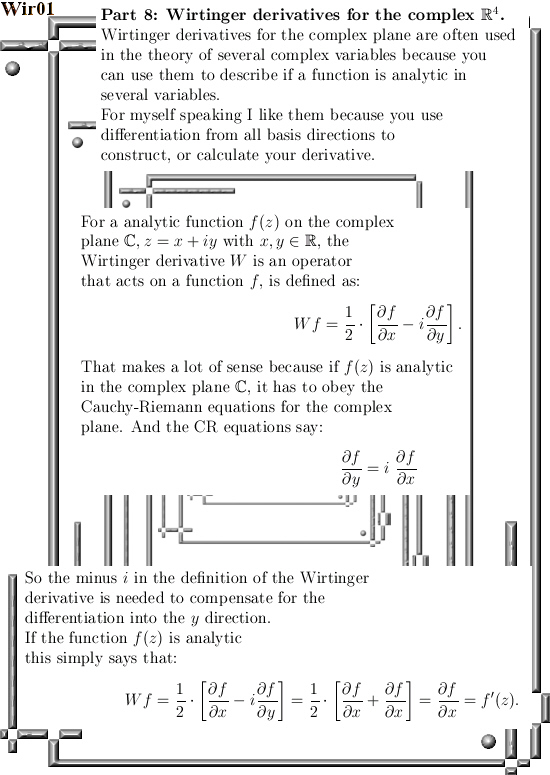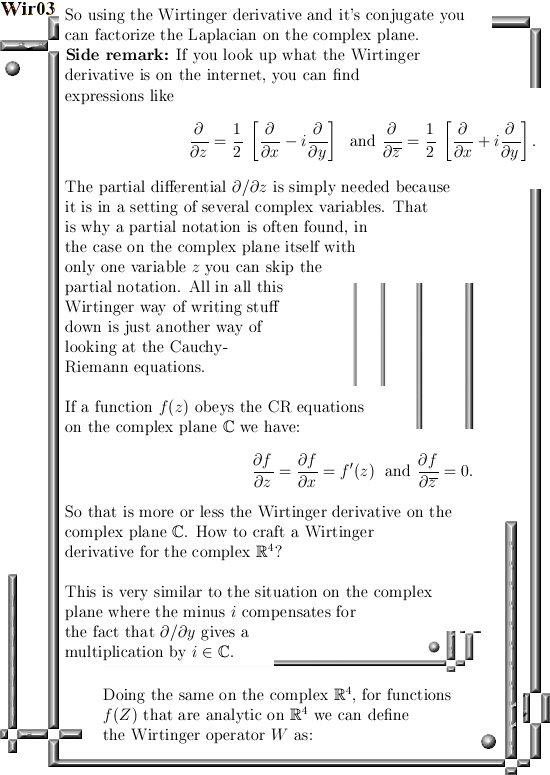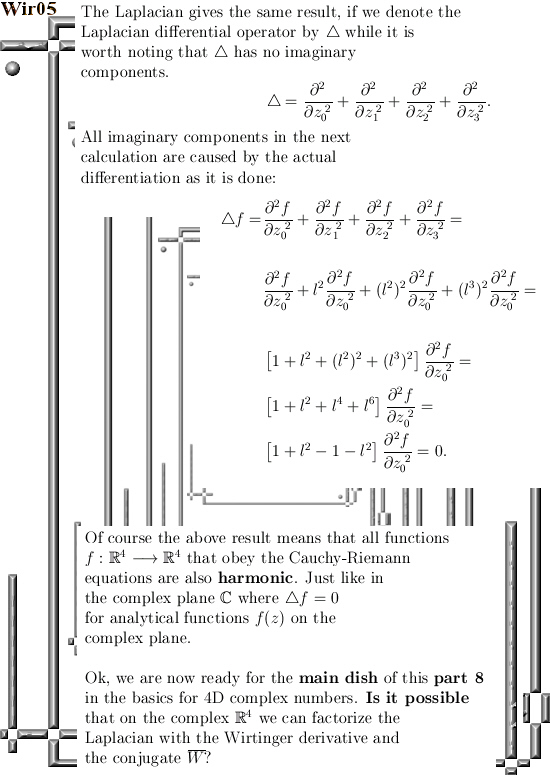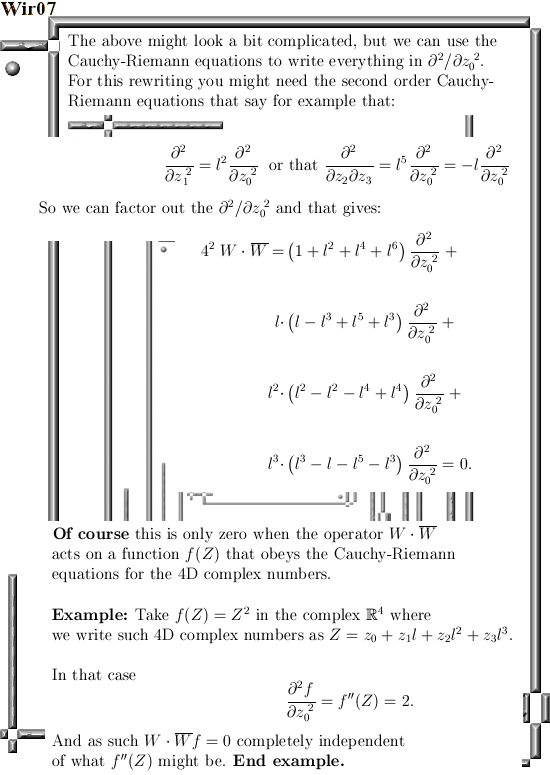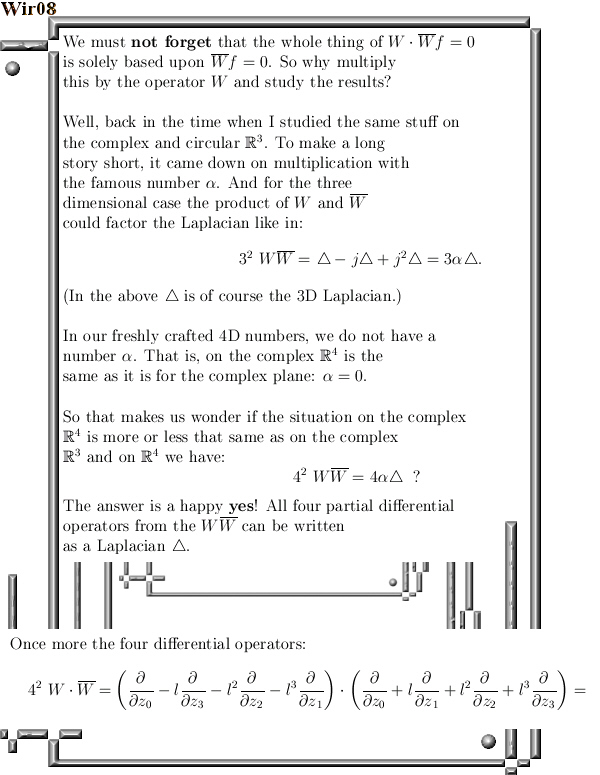Further reading from a wiki (of course that is only about 2D complex numbers from the complex plane):
Wirtinger derivatives
https://en.wikipedia.org/wiki/Wirtinger_derivatives

Ok, that was it. Till updates.

# The basics of 4D complex numbers.

In the previous post on 4D complex numbers I went a little bit philosophical with asking if these form of crafting a 4D number system is not some advanced way of fooling yourself because your new 4D thing is just a complex plane in disguise…

And I said let’s first craft the Cauchy-Riemann equations for the 4D complex numbers, that might bring a little bit more courage and making us a little bit less hesitant against accepting the 4D complex numbers.

In this post we also do the CR equations and indeed they say that for functions like f(Z) = Z^2 you can find a derivative f'(Z) = 2Z. So from the viewpoint of differentiation and integration we are in a far better spot compared to the four dimensional quaternions from Hamilton. But the fact that the CR equations can be crafted is because the 4D complex numbers commute, that is XY = YX. And on the quaternions you cannot differentiate properly because they do not commute.

So crafting Cauchy-Riemann equations can be done, but it does not solve the problem of may be you are fooling yourself in a complicated manner. Therefore I also included the four coordinate functions of the exponential 4D curve that we looked at in the previous post.

All math loving folks are invited to find the four coordinate functions for themselves, in the next post we will go through all details. And once you understand the details that say the 4D exponential curve is just a product of two exponential circles as found inside our 4D complex numbers, that will convince you much much more about the existence of our freshly unearthed 4D complex numbers.

Of course the mathematical community will do once more in what they are best: ignore all things Reinko Venema related, look the other way, ask for more funding and so on and so on. In my life and life experiences not one university person has ever made a positive difference, all those people are only occupied with how important they are and that’s it. Being mathematical creative is not very high on the list of priorities over there, only conform to a relatively low standard of ‘common talk’ is acceptable behavior…

After having said that, this post is partitioned into five parts and is 10 pictures long. It is relatively basic and in case that for example you have never looked at matrix representations of complex numbers of any dimension, please give it a good thought.

Because in my file I also encountered a few of those professional math professors that were rather surprised by just how a 3 by 3 matrix looks for 3D complex numbers. How can you find that they asked, but it is fucking elementary linear algebra and sometimes I think these people do not understand what is in their own curriculum…

Ok, here are the 10 pictures covering the basic details of 4D complex numbers: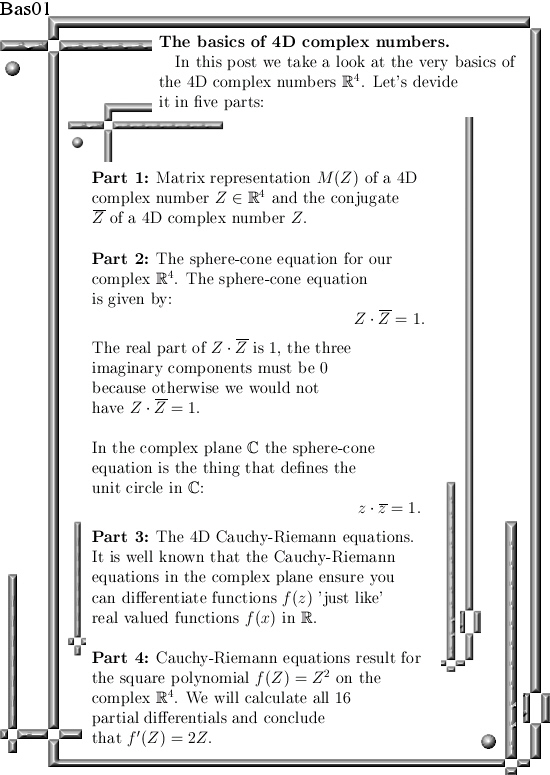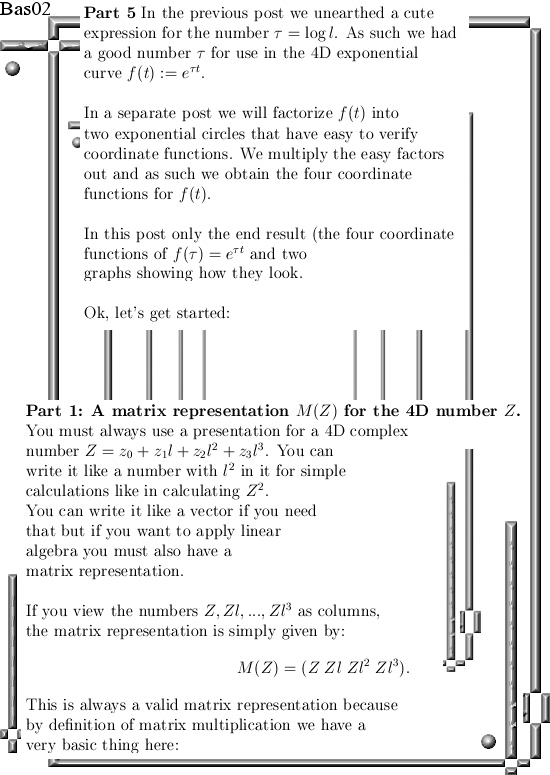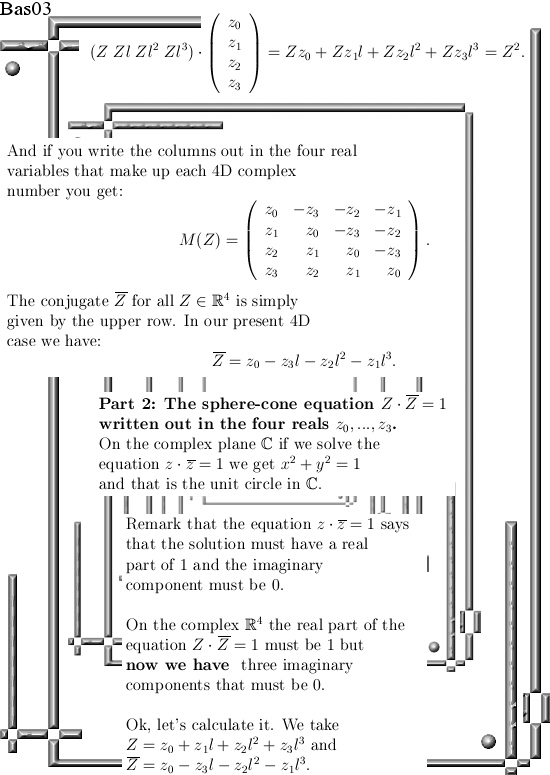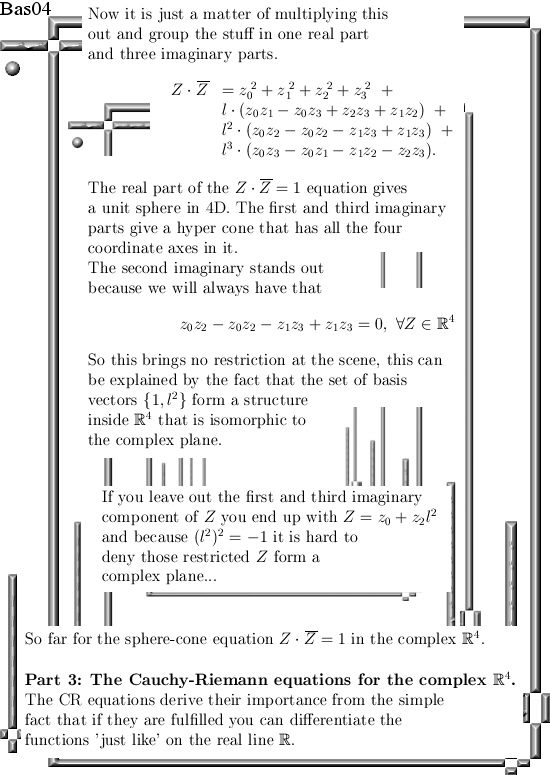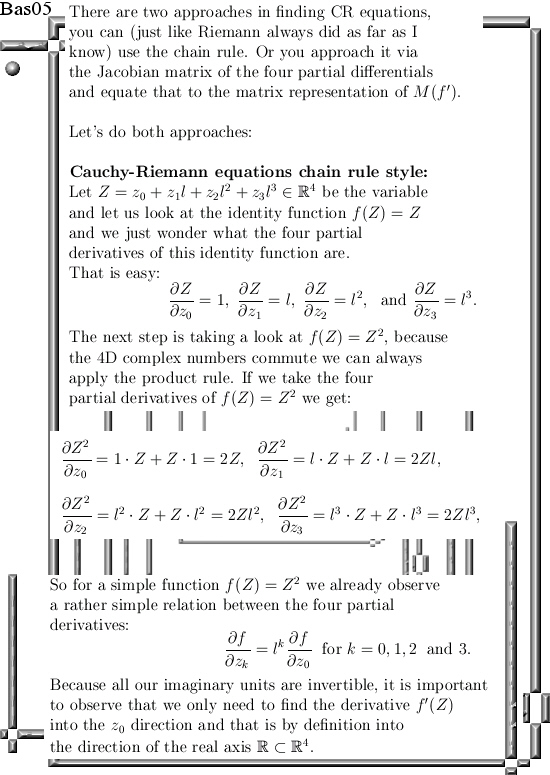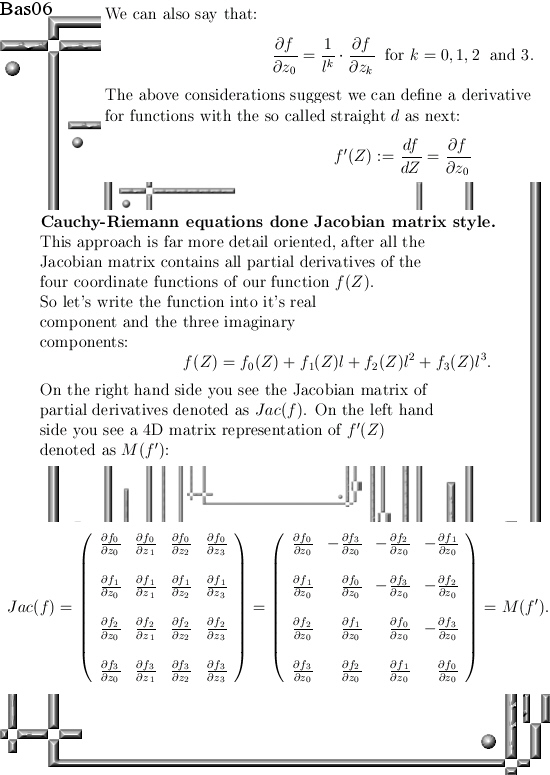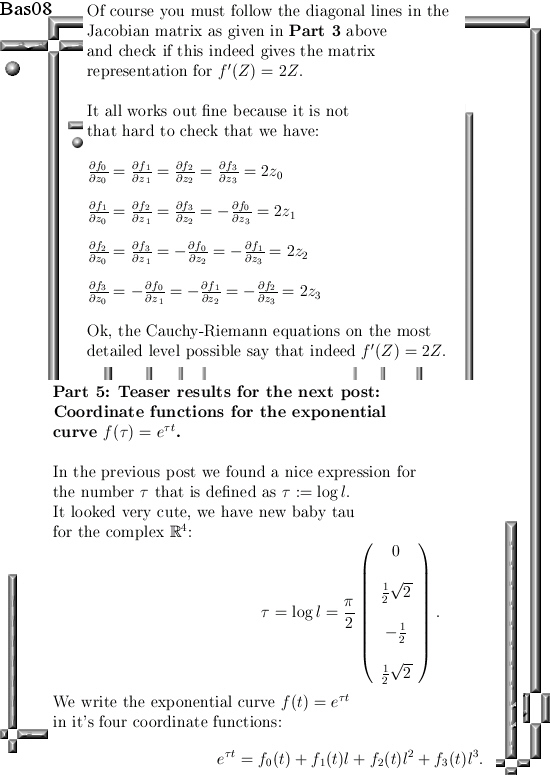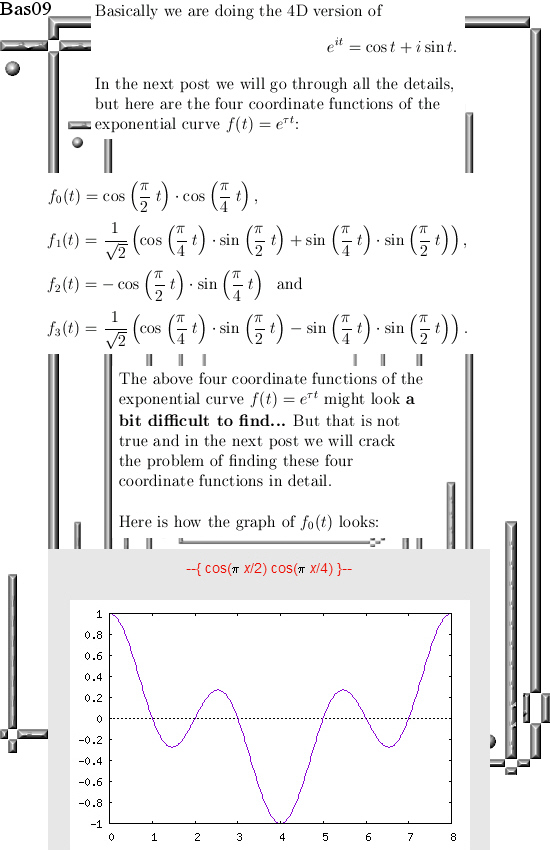Ok, that was the math for this post.

And may be I am coming a bit too hard on the professional math professors. After all they must give lectures, they must attend meetings where all kinds of important stuff has to be discussed until everybody is exhausted, they must be available for students with the questions and problems they have, they must do this and must do that.

At the end of the day, or at the end of the working week, how much hours could they do in free thinking? Not that much I just guess…

Let’s leave it with that, see you in the next post.

# Curl, curl and more curl.

This post contains seven examples of the differential operator named curl. The motivation for this update lies in the fact that after my humble opinion inside the set of equations known as the Maxwell equations there is a tiny fault: Rather likely electrons carry at least a net magnetic charge.
And because they carry net magnetic charge they not only accelerated by electrical fields but also magnetic field.

If you have an old television set with one of those fancy tubes that contain one electron cannon for a black & white television and three electron cannons for a color television. With the help of a stack of these strong neodymium magnets it is easy to give experimental proof that the electrons indeed get accelerated… Why in the course of over one hundred years not one of the professional physics professors has done this is unknown to me; may be it is the separation of ‘theoretical professors’ versus ‘experimental ones’ a reason for this omission. May be it is the use of dogma (unproven things that live inside a belief system, in this case the belief system that magnetic monopoles do not exist).

__________

Anyway the Maxwell equations contain a lot of the curl operator, that is not needed per sé but it makes the formulae look sleek and short. Originally Maxwell had a set of like 20 equations or so while at present day only four remain. But if you see those four equations for the first time it is very impressive, only over time you get used to it.

This post is 13 pictures long, size 550 x 775 pixels.
I start with examples that are as simple as possible and very slowly bring in a bit more abstraction.
Therefore I hope it is very readable, have fun with it!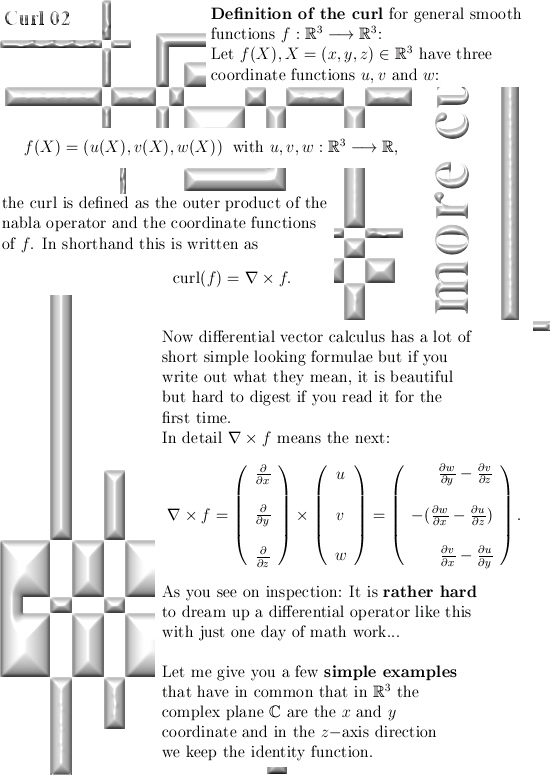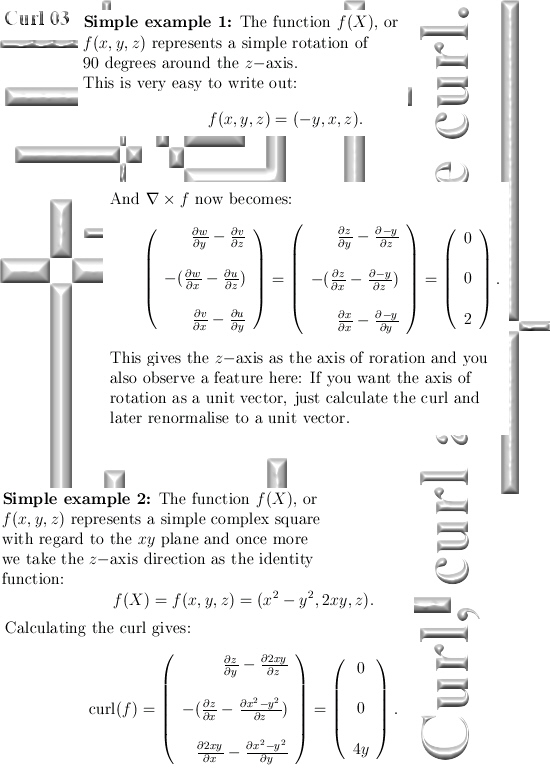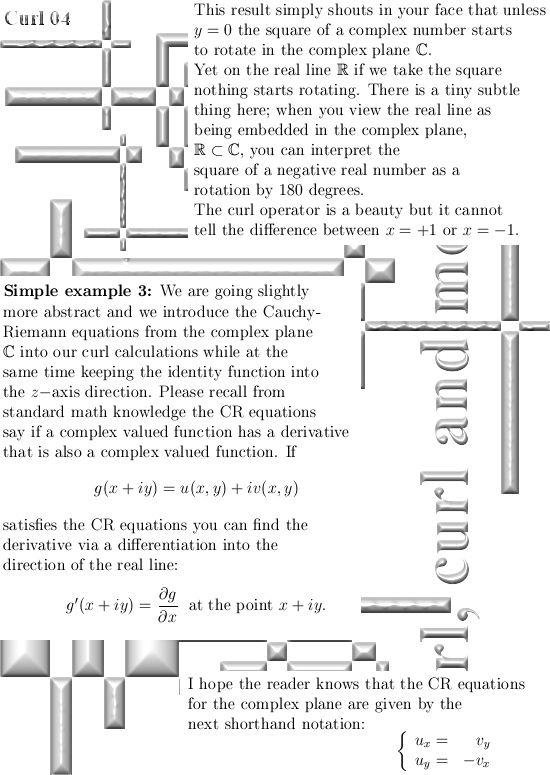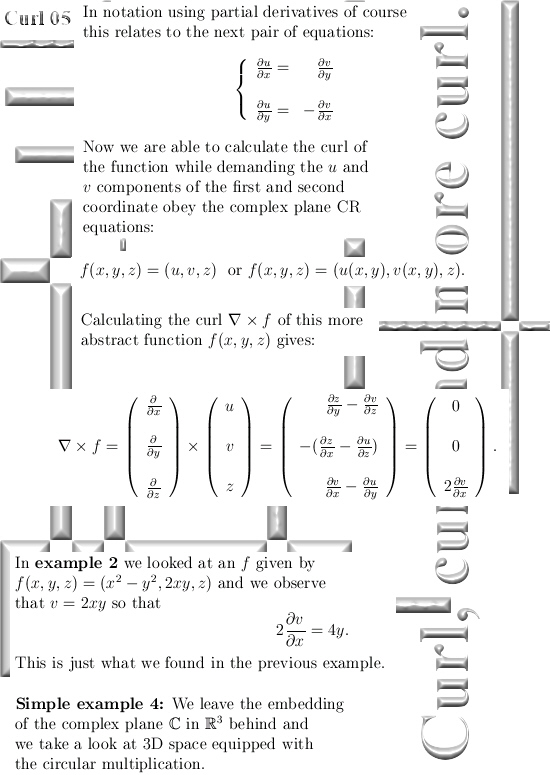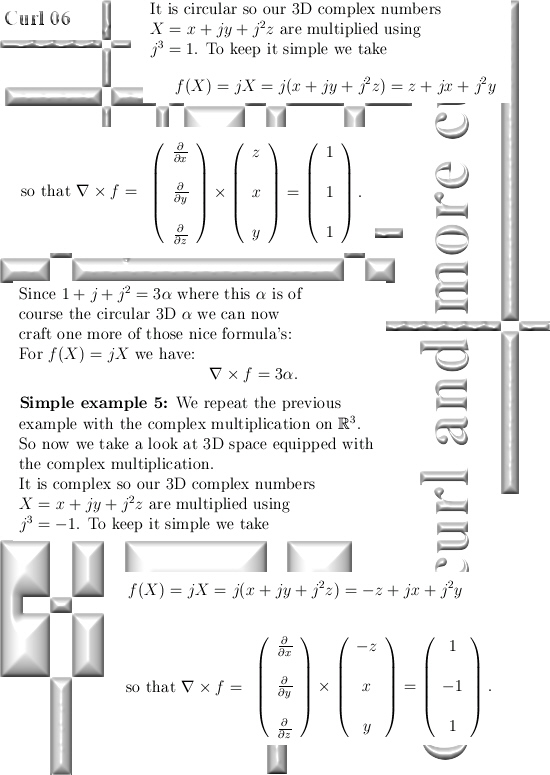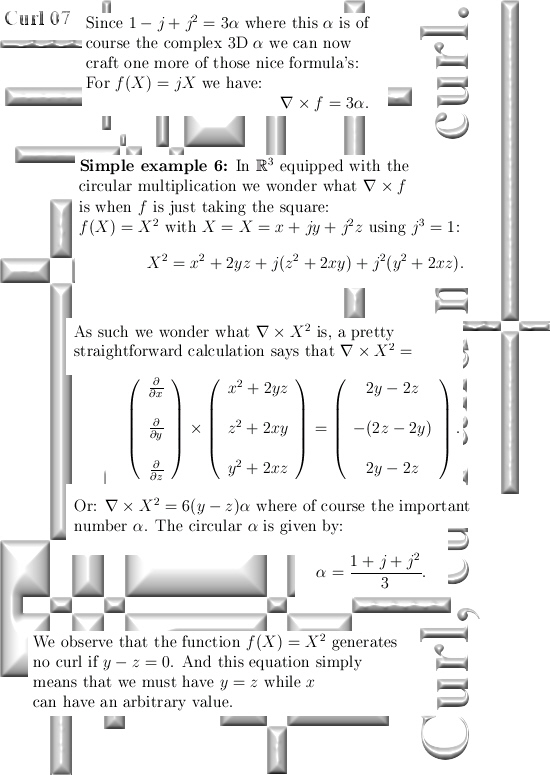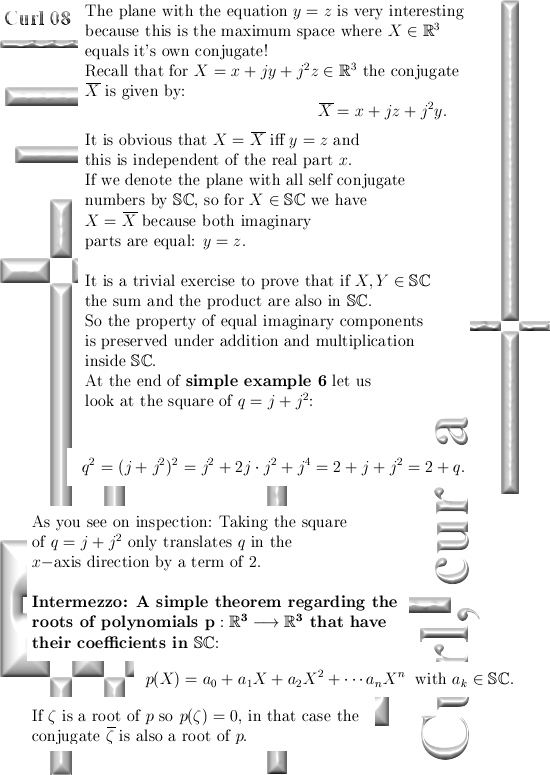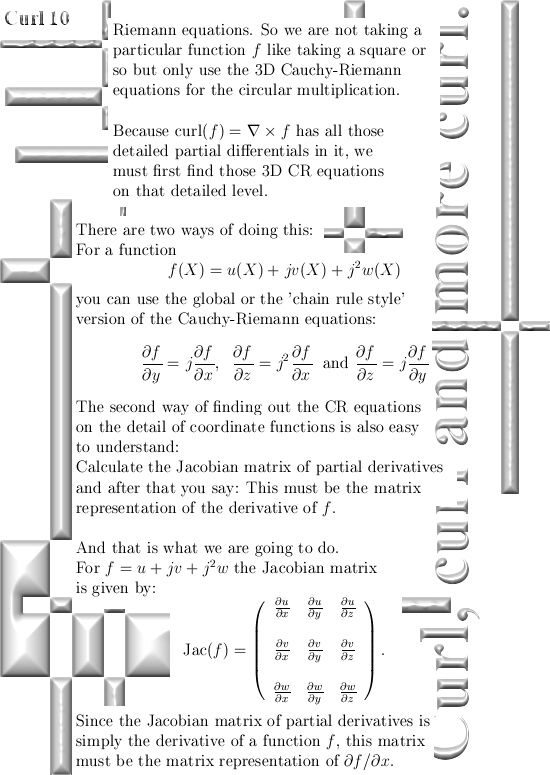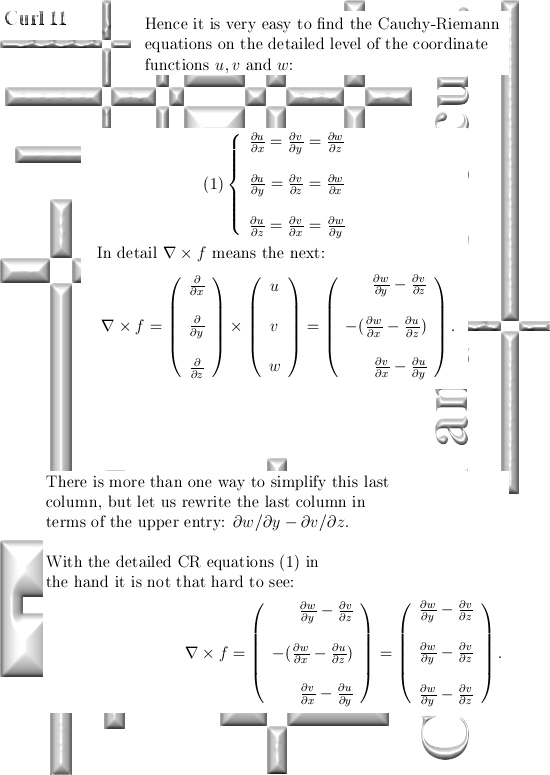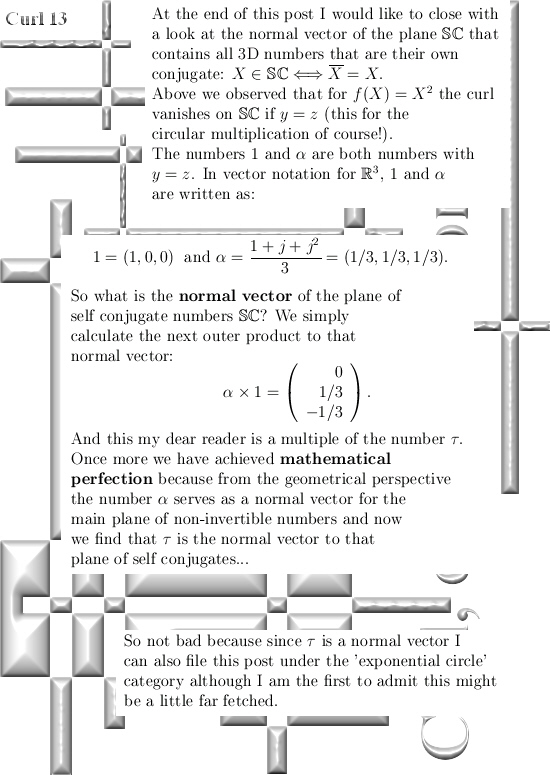As far as I can remember, in the first year I opened these investigations into higher dimensional number systems again I calculated the curl for the complex multiplication in 3D space. In this post we only looked at the circular version of stuff.

But I can´t find it back so I cannot place a hyperlink to it.

Anyway, here is a nice wiki with the curl expressed as an integral (often much harder to calculate but nice to observe it can also be done that way):

Curl (mathematics)
https://en.wikipedia.org/wiki/Curl_(mathematics)

And because this post was motivated by all that curl in the Maxwell equations, I tried to find back when I originally started writing about electrons having magnetic charge instead of being magnetic dipoles like they are tiny bar magnets. It was 29 April that inside the math pages I found the first update on that. Here it is:

From 29 April 2014 : Do electrons have spin?
http://kinkytshirts.nl/rootdirectory/just_some_math/3d_complex_stuff02.htm#29April2014

# Teaser picture for a new update on differential equations.

I am going to make this update on the other website so in about one week you can check it out on page 4 of the 3D complex numbers. I admit I have been lazy the last month but now I have stopped smoking over three years my health is still improving so I am more exploring the environment with my bike while I can do that again…

Ha! At my worst about four years back I could only walk 100 to 125 steps and after that I needed to pause 3 to 4 minutes because I got camps in one of my legs. So it finally dawned on me I had to stop this nicotine addiction because the next phase would be a wheelchair combined with an oxygen mask and a tank of high pressured liquid oxygen on the back of my wheelchair.

Looking back I am glad I got so ill because without it I would never have managed to stop smoking those ridiculous amounts of cigarettes day in day out.

Sorry to bother you with my past health problems, this new teaser picture is rather funny and I hope also intriguing: I have crafted three coordinate functions x(t), y(t) and z(t) and if you differentiate them with respect to the time t you get a 3D square of the vector (x(t), y(t), z(t)).

So check it out for yourself; take the derivative of the three coordinate functions and see if you can get the three equations as on the bottom of the teaser picture…

The new update (on the other website) is ten pages long meaning it is 10 pictures of size 550 x 1100. Click on the teaser picture to land on the new update:For me it is just so cute: If you differentiate to time you get the square of the position you are in the 3D complex number system.

For use in the science of physics I do not think it is that important because real physical problems never rely on the coordinate system you use, but you never know…
For use in the science of math it is also not important because professional math professors still have not developed the cognitive capabilities of understanding 3D complex numbers.

I also made a teaser picture for use on the other website, it is the same solution to the simple differential equation as above but this time I solved it inside the complex plane. Of course I could not use that on this website as the first teaser picture given the face we more or less always try to focus on the 3D complex & circular number systems…Ok, end of this post.
And life, as usual life will go on.

# Wirtinger derivatives and the factorization of the Laplacian.

This post could have many titles, for example ‘Factorization of the Laplacian using second order Cauchy-Riemann equations’ would also cover what we will read in the next seven pictures.

The calculation as shown below is, as far as I am concerned, definitely in the top ten of results relating to all things 3D complex numbers. Only when I stumbled on this a few years back I finally understood the importance of the so called sphere-cone equation.

The calculation below is basically what you do when writing out the sphere-cone equation only now it is not with variables like x, y and z but with the partial differential operators with respect to x, y and z. In simplifying the expressions we get I use so called second order Cauchy-Riemann equations, if you understand the standard CR equations these second order equations are relatively easy to digest.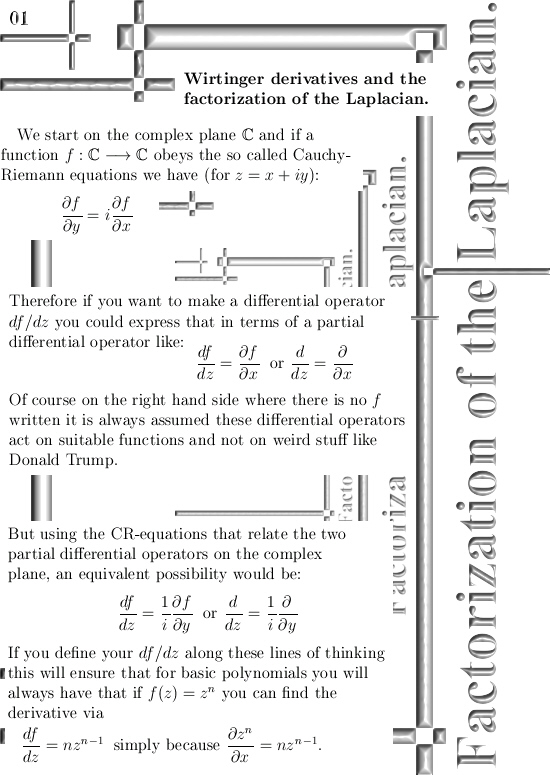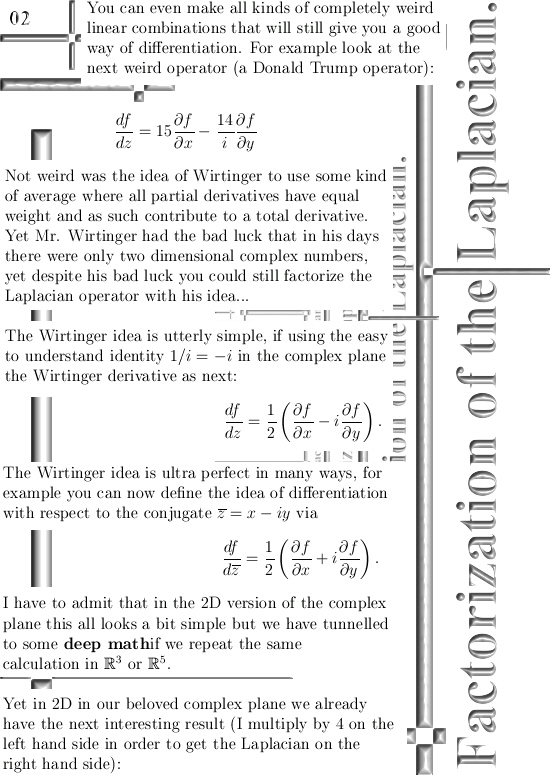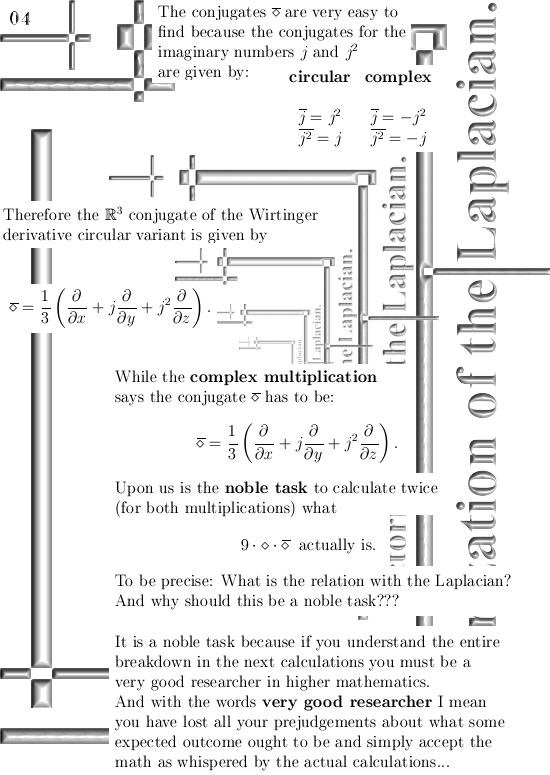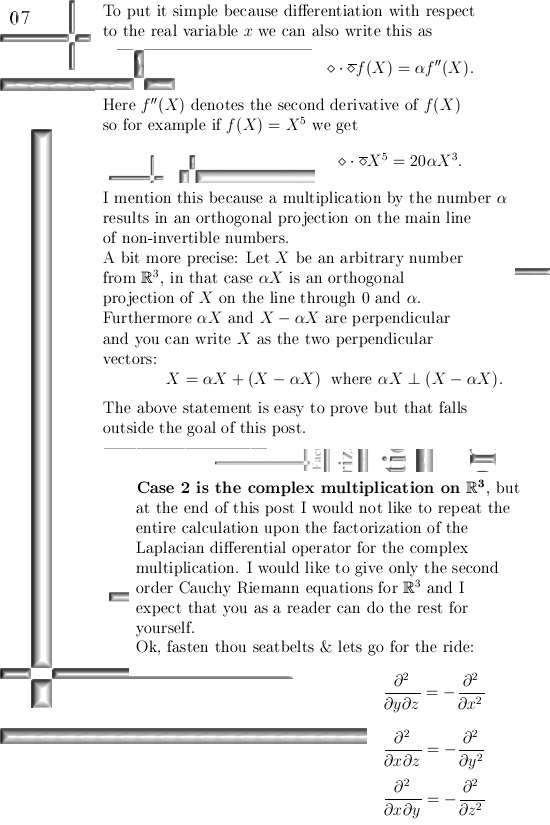This post is also categorized under Quantum Mechanics, the reason for that is that the wave equation contains the Laplacian operator and the more you know about that rather abstract thing the better it is in my view.

I would like to close with a link to a wiki on Wirtinger derivatives, originally they come from theory with several complex variables. That explains why in the wiki the Wirtinger derivatives are written as partial derivatives while above we can use the straight d´s for our differential of f.

Here is the wiki: Wirtinger derivatives
https://en.wikipedia.org/wiki/Wirtinger_derivatives

# Calculating the Laplacian using the Cauchy-Riemann equations.

Without doubt the Laplacian is a very important differential operator. It plays a major role in for example the classical wave equation and also the Schrödinger wave equation from quantum mechanics.

Now scroll a bit back until you find the post on the Cauchy-Riemann equations, at the end I used the phrase ‘Cauchy-Riemann equations chain rule style’ and this is how we can crack in a very easy way how the Laplacian operates on functions that obey the CR equations on 3D complex numbers.

I have hundreds and hundreds of pages of math stuff on the 3D complex number system and very often I use the number alpha. This number alpha is so important, not only in 3D, that it is worth to post a few posts on them.

For the time being, I just conducted a simple Google search on the phrase ‘3d complex numbers’ in the search detail for pictures. And every time this old teaser picture from the other website pops up: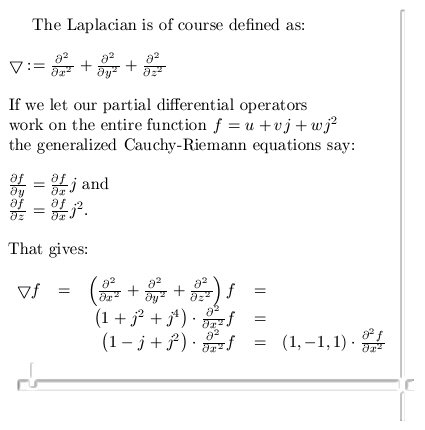At the end you see that (1, -1, 1), well that is three times alpha.

It is a nice exercise to prove that the square of alpha equals alpha.
So alpha is in the same category as for example numbers like 0 and 1 because if you square those you also get the original number back in return.

After all one squared equals one and zero squared equals zero.

End of this update, till updates.

# And now we are with three…

In Oct 1990 (estimated) I found the 3D complex numbers, a few years back I discovered that a guy named Dennis Morris has found them too. Dennis even wrote a book about it, this book was published twice and I was able to get a hand on the second publication.

For normal humans the book from Dennis is a good starter, for me it has the depth of a bird bath.

We do not complain, today on the preprint archive I found the next pdf file:

On a novel 3D hypercomplex number system
http://arxiv.org/pdf/1509.01459v1.pdf

And this work has not the depth of a bird bath, it is much more the depth of a human bathtub.
There ar some dumb typo’s, for example table 1 contains a very stupid error so that has to be corrected.

The writer of the preprint article goes under the name of Shlomo Jacobi and since his residence was Israel we might jump to the conclusion he was a Jew. Let religion be no problem because after all the Muslims were once far ahead of the Western powers but because one of their religious leaders declared math as being from the devil, Muslims find themselves at the receiving end of military powers for about one thousand years…

Now back to our Jewish pdf file: Table 1 should be corrected and I, Reinko Venema, I give them a big applause because if you scroll down to page 39 you observe they have found the 3D exponential circle too!

Well I have found all exponential curves in all possible dimensions, so I am very pleased to invite the Jewish mathematical community upon further investigations into this math detail.

End of this update, till updates.

# Cauchy-Riemann equations for the complex plane and for 3D complex numbers.

In itself the name of ‘Cauchy-Riemann equations’ is a terrible way of naming these equations because it says nothing about why they are important.

It would be better to name the stuff involved like ‘Chain rule equations for partial derivatives’ because if that would be the case you would understand why these equations are worth your precious time anyway…

This update is 8 pictures of size 550 by 550 pixels or about 5 pages of A4 size if crafted in the A4 size format.
Now why are CR equations important?
Very simple: You can find the derivative of a function just like on the real number system or in the complex plane. That is why CR equations are the basic food for understanding higher dimensional complex number systems…

Enough of the bla bla, here are the 8 pictures: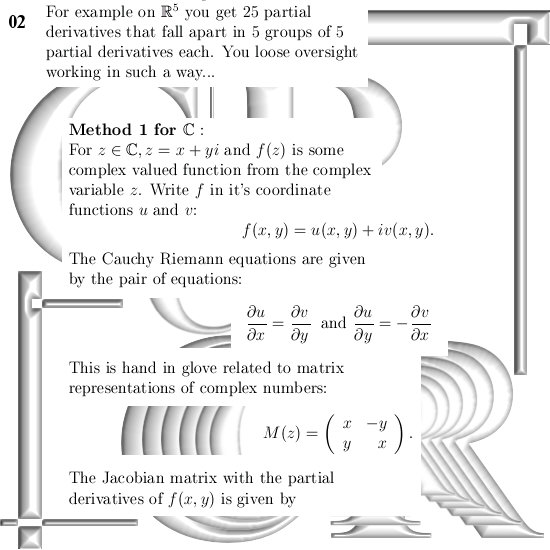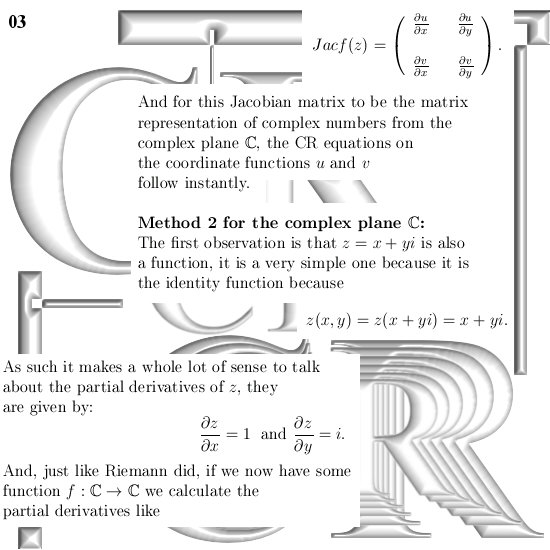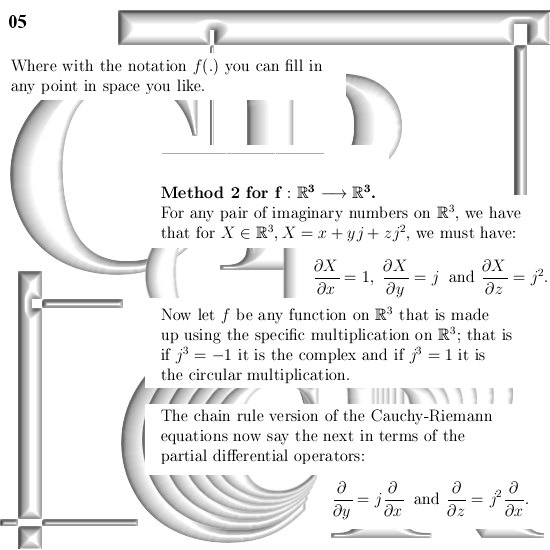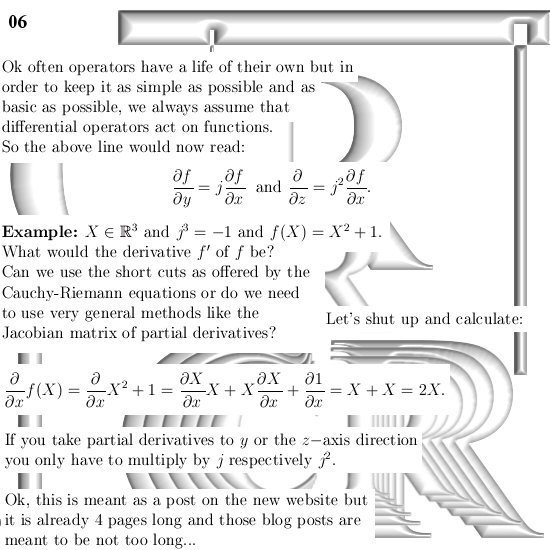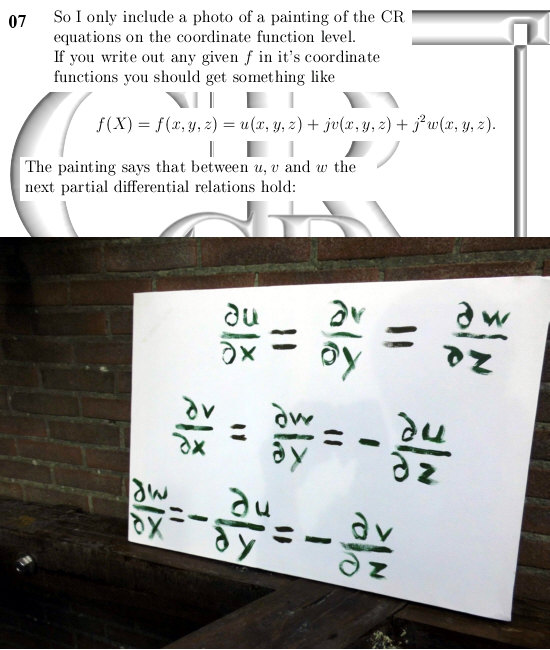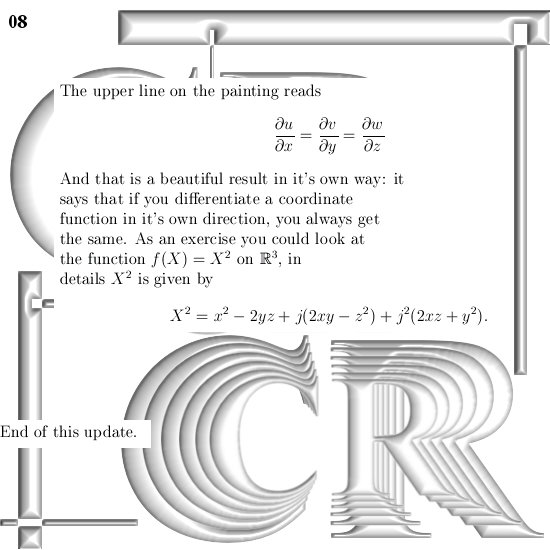This stuff is basic stuff so it should be hanging out on this new website.